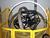## Converting Float to packedTags:
RPG
I am trying to convert a Float8 to a 17 6 Packed and I am loosing 1/100000th of the value of the decimal portion. The packed result is 1/100000th less than the Float value. How do I stop this from happening? I am calculating taxes.

Thanks. We'll let you know when a new response is added.

How are you attempting the conversion? Are you using the MOVE opcode, or the %dec BIF? Could this be a truncation matter … perhaps all you need is an eval(h)?

A code snippet would help diagnose what’s happening.

Good luck,

## Discuss This Question: 8 Replies

Thanks. We'll let you know when a new response is added.
• I had the same problem but going in the other direction. I solved it with the following: Eval hfloat = decfield * 1.000005 I had to experiment to fing the right number of zeros, but it finally worked.
report
•You don't "solve" it. Binary representations of fractional decimal values often are only approximations because that's how fractional values work when converting from one number base to another with a fixed number of digits. Simply test it by manually converting .03 decimal to binary and back again. Try with 1-byte, 2-byte, 4-byte and 8-byte binary variables. There will always be a loss of same small fraction. That is exactly why formats such as packed-"decimal" and binary-coded-decimal were invented in the first place. A value such as .03 cannot be accurately represented in binary form with an infinite number of bytes. Don't use binary data types (e.g., floating point) for fractional decimal calculations. Alternatively, don't use fractional values -- always convert to integers. For example, don't store dollars as packed (9,2). Instead, store as pennies with packed (9,0). The same digits are used, but there are no fractional portions. Do it all with integer math. Of course, when you do that, you introduce multiplication by and division by 100, which tends to offset any advantage of using floating-point in the first place. Tom
report
•Typo -- "without an infinite number of bytes..." Tom
report
• I understand the lack of precision using floating point numbers, but why does an 8F number = 1.929000000000E+001convert to 15P5 value of 19.28999? My instruction is 15P5 = 8F.
report
•When I use SQL to insert data into FLD05F,

INSERT INTO DECTEST VALUES(1.9290000E+002)

the floating point field contains data that can be off by one digit.

It's not the conversion to 15P5, it's already messed up in the float field.

FLD05F

19290000.E-001

19290000.E-002
19290000.E-003
19290000.E-004
19289999.E-005
19290001.E-006
19290000.E-007
19290000.E-008
19290000.E-003

19290000.E-007
19289999.E-005
19289999.E-005

report
•I probably should have named that field FLD08F - as it's 8 Float.
report
• The data comes to me in an Excel spreadsheet. I have elected to use Scott Klement's XLPARSER4 service program to extract the data, so I am stuck with dealing with floating point numbers.

You say the float field is already messed up. Why does 1.92900000000.E+001 come out as anything but 19.29? I know it does, because a 15P5 field shows it as 19.28999, and a 12P2 field shows it as 19.28.

The Excel column in question is an item cost field with two decimal places. Can I be sure of getting the same number is I use 'eval (h) 15P5 = 8F'?
report
•Assuming that you can't get the XLPARSER4 to pull the field down as text ..

There is something about Half adjust EVAL(H) .. I will have to think on this... got it .. there has to be one more digit (6) in the float then the 15P5

so this 19289999.E-005 goes to 192.89999

works for 19290001.E-006 which goes to 19.29000

report